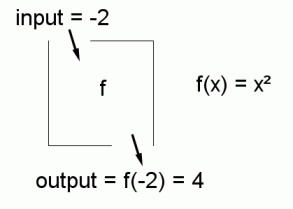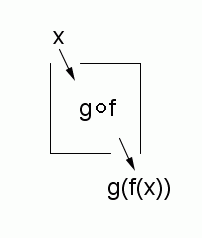﻿ Composition of Functions
COMPOSITION OF FUNCTIONS
by Dr. Carol JVF Burns (website creator)
Follow along with the highlighted text while you listen!

Numbers can be ‘connected’ to get new numbers: for example, $\,1+2=3\,$.
Indeed, addition ‘$\,+\,$’ is a connective for numbers.

Sets can be ‘connected’ to get new sets: for example, $\,\{1\} \cup \{2\}=\{1,2\}\,$.
Indeed, the union operator ‘$\,\cup\,$’ is a connective for sets.

Functions can also be ‘connected’ to get new functions:
the most important way to do this, called function composition, is the subject of this section.

First, some basic review.
A function is a rule that takes an input, does something to it, and gives a unique corresponding output.
If the function name is $\,f\,$, and the input name is $\,x\,$, then the unique corresponding output is called $\,f(x)\,$ (which is read as ‘$\,f\,$ of $\,x\,$’).
Note that $\,f\,$ and $\,f(x)\,$ are different mathematical expressions:
$f\,$ is the name of the function;
$f(x)\,$ is the output from the function $\,f\,$ when the input is $\,x\,$.

It is often helpful to think of a function as a ‘box’.
You drop an input in the top, something happens to the input inside the box, and the output drops out the bottom.
The box is labeled with the name of the function.Constructing a Composition of Functions To construct a composition of functions, start by putting function boxes in sequence, (i.e., one right after the other), as shown at right. An input $\,x\,$ is dropped into the first box (call it $\,f\,$), giving the output $\,f(x)\,$. This output $\,f(x)\,$ is then dropped into the next box (call it $\,g\,$), giving $\,g(f(x))\,$. Now, bundle these two functions $\,f\,$ and $\,g\,$ into a single, new, function: this new function takes an input $\,x\,$ and gives the output $\,g(f(x))\,$. What should this new function be named? Certainly, the name should have something to do with both $\,f\,$ and $\,g\,$. It can't be named $\,fg\,$ or $\,gf\,$, because these names are already taken to mean multiplication of outputs: for example, $\,(fg)(x) := f(x)g(x)\,$. (Remember that ‘$\,:=\,$’ means ‘equals, by definition’.) In whatever name is decided upon, it might make sense to put the letter $\,f\,$ before the letter $\,g\,$, since the function $\,f\,$ gets to act on the input first. On the other hand, it might make sense to put the $\,g\,$ first, since the $\,g\,$ comes first when you look at the final output, $\,g(f(x))\,$. Decisions, decisions, decisions!The name that was decided upon is: $\,g\circ f\,$ For us (high school mathematics and most others), the name chosen for this new function is $\,g\circ f\,$, which is read aloud as ‘$\,g\,$ circle $\,f\,$’ or ‘$\,g\,$ composed with $\,f\,$’. That is, by definition: $(g\circ f)(x) := g(f(x))$ This sentence is read aloud as: “$\,g\,$ circle $\,f\,$ of $\,x\,$ equals $\,g\,$ of $\,f\,$ of $\,x\,$” A function like $\,g\circ f\,$ is called a composite function. Notice that $\,g\circ f\,$ is the name of our ‘new’ function; whereas $\,(g\circ f)(x)\,$ is the output from the function $\,g\circ f\,$ when the input is $\,x\,$. One thing that is a bit different is that $\,g\circ f\,$ is a pretty long function name. It has several ‘pieces’ to it. It's certainly not as simple a name as a plain 'ole single-letter $\,f\,$ or $\,g\,$. To keep this long function name together, you must put the name inside parentheses: that is, notice that we said $\,(g\circ f)(x)\,$, not $\,g\circ f(x)\,$. BE CAREFUL!! For the function $\,g\circ f\,$, the function $\,f\,$ acts first, and the function $\,g\,$ acts last. The letter $\,g\,$ certainly appears first in the name, but it doesn't get to act on the input first. That is, in the output $\,g(f(x))\,$, the function $\,f\,$ is closest to the input $\,x\,$, so it acts first.Practice with function composition

Let $\,f(x) = x^2\,$ and $\,g(x) = 2x\,$.

Then,

 $\,(g\circ f)(x)$ $:=$ $g(f(x))$ definition of the function $\,g\circ f$ $=$ $g(x^2)$ work from the inside to the outside; substitute $\,x^2\,$ for $\,f(x)\,$ $=$ $2x^2$ apply the ‘multiply by $\,2\,$’ function, $\,g\,$, to $\,x^2\,$

Also,

 $(f\circ g)(x)$ $:=$ $f(g(x))$ definition of the function $\,f\circ g$ $=$ $f(2x)$ work from the inside to the outside; substitute $\,2x\,$ for $\,g(x)\,$ $=$ $(2x)^2$ apply the squaring function, $\,f\,$, to $\,2x\,$ $=$ $4x^2$ simplify

Notice that $\,g(f(x))\,$ is not the same as $\,f(g(x))\,$.
In general, function composition is not commutative.

Master the ideas from this section